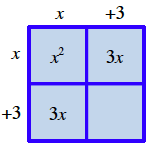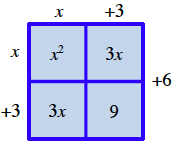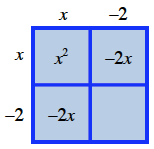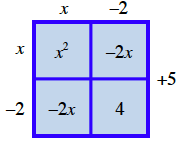### Home > INT3 > Chapter 3 > Lesson 3.1.1 > Problem3-13

3-13.

For each quadratic function below use the method of completing the square or averaging the $x$-intercepts to rewrite the equation in graphing form. Then, state the line of symmetry and give the vertex of each parabola. Try to use each method at least once.

1. $f ( x ) = x ^ { 2 } + 6 x + 15$

How many ones do you need to make a perfect square? How many do you have?

2 by 2 square, labeled as follows: top edge left, x, top edge right, + 3, left edge top, x, left edge bottom, + 3, interiors: top left, x square, top right, 3, x, bottom left, 3, x.

Added to square: interior bottom right, 9, right edge, + 6.

$f(x)=(x+3)^2+6$
vertex: $(−3,6)$
axis of symmetry: $x=−3$2. $y = x ^ { 2 } - 4 x + 9$

2 by 2 square, labeled as follows: top edge left, x, top edge right, minus 2, left edge top, x, left edge bottom, minus 2, interiors: top left, x square, top right, negative 2, x, bottom left, negative 2, x.

Added to square; interior bottom right, 4, right edge, + 5.3. $f ( x ) = x ^ { 2 } - 8 x$

Follow the hints in parts (a) and (b).

4. $y = x ^ { 2 } + 7 x - 2$

$y=(x+3.5)^2−14.25$